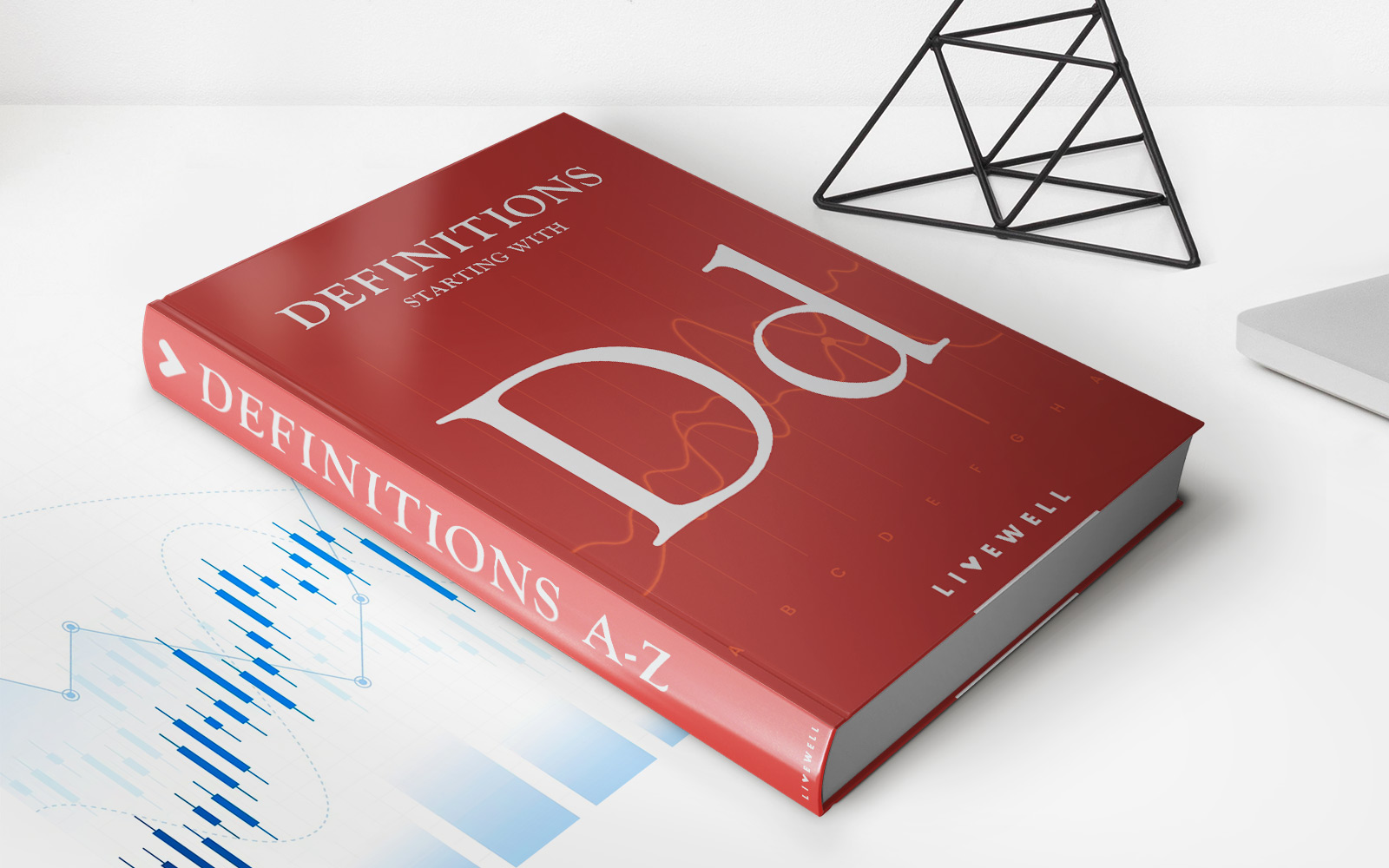Home>Finance>What Is A Decile? Definition, Formula To Calculate, And ExampleFinance

# What Is A Decile? Definition, Formula To Calculate, And Example

Discover the definition and formula to calculate deciles in finance, along with a helpful example. Master the concept of deciles in just a few simple steps.

## Understanding Deciles in Finance

Welcome to the world of finance, where numbers rule and calculations reign supreme. In this complex landscape, one term that often pops up is “decile.” But what exactly is a decile and how does it relate to financial analysis? In this article, we will unravel the mystery behind deciles, exploring their definition, formula for calculation, and providing a practical example.

## Key Takeaways:

• A decile is a statistical measure that divides a particular set of data into ten equal parts.
• Deciles are commonly used in finance to analyze the distribution of individuals or investments within a specific population.

## Defining Deciles

A decile is a statistical tool used to evaluate the distribution of data. It splits a given dataset into ten equal parts, with each part representing 10% of the total population.

Deciles are especially useful in finance as they help identify where entities or individuals fall in terms of wealth, income, or performance relative to the entire population. This segmentation allows for a more detailed analysis of how resources are distributed among different groups.

## Calculating Deciles

To calculate a decile, you need to follow a simple formula:

Decile = (n/10) * i

In this formula, n represents the total number of individuals or investments, and i denotes the desired decile position (ranging from 1 to 10).

Let’s say you have a dataset of 100 investments, and you want to find the 5th decile. Using the formula, you would calculate it as follows:

Decile = (100/10) * 5 = 50

This calculation indicates that the 5th decile represents the 50th highest-performing investment in the dataset.

## An Example of Deciles in Finance

To illustrate the practicality of deciles in finance, let’s consider a hypothetical scenario. Imagine you are a portfolio manager responsible for overseeing a group of 1,000 stocks. By analyzing the deciles of these stocks’ performances, you can gain valuable insights into their distribution and ranking within the overall portfolio.

After calculating the deciles for your stock portfolio, you discover that the top decile (decile 10) comprises the 100 best-performing stocks, indicating a high level of success. On the other hand, the bottom decile (decile 1) consists of the 100 worst-performing stocks, highlighting potential areas for improvement.

By further analyzing these deciles, you can dig deeper into the performance of individual stocks within each group, pinpointing specific areas that drive success or identifying potential challenges that need to be addressed.

## Wrapping Up

Deciles play a crucial role in finance, enabling a more in-depth analysis of how data is distributed within a particular population. By categorizing data into ten equal parts, deciles provide a comprehensive understanding of performance, wealth distribution, or any other variable of interest.

Remember, understanding how to calculate deciles and interpret their results can provide valuable insights for decision-making in finance. So next time you come across the term “decile,” you’ll know exactly what it means and how it can impact your financial analysis.

### Related Post

• https://livewell.com/finance/what-is-a-decile-definition-formula-to-calculate-and-example/